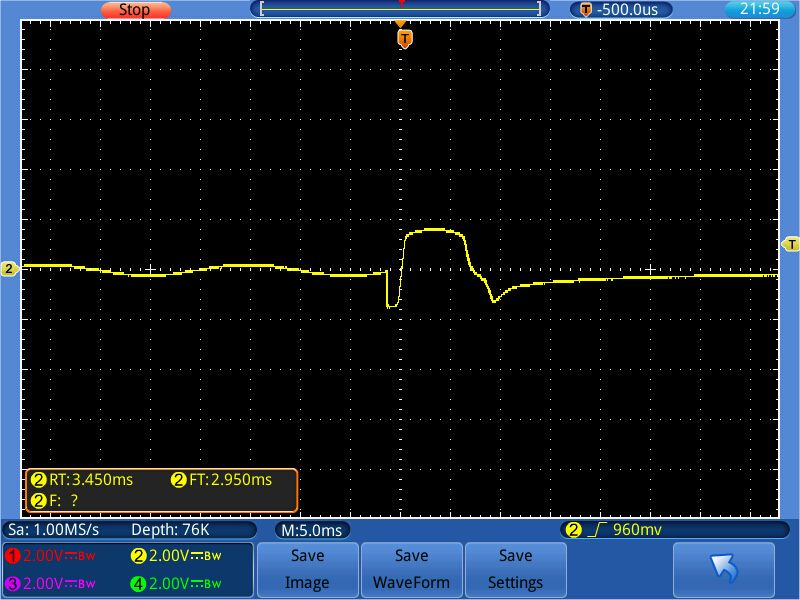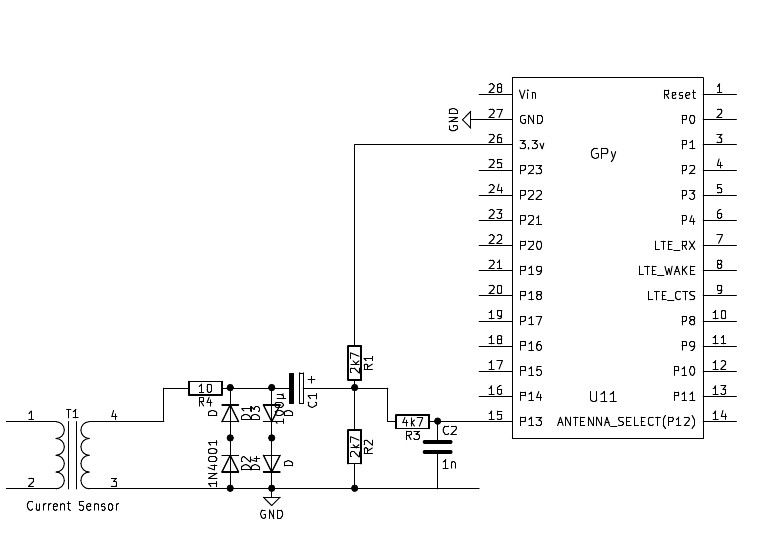# STC013-015 analogic schema at 3.3 v

• Hello,

I’ve a sensor STC013-015 AC 15A, please , I need the elettronic schema , for analogic connection to Lopy at 3.3 volt in out.

Stefano

• The Stc013 sensor has an electronic circuit on board clamp with resistor and diodes

I looked up the sensor data sheet. If your sensor has printed on the case 15A/1V, then it is the voltage type sensor. And That's the one you need. But I made my tests with exactly that SCT013-015 sensor, and got up to 15Vpp, when there was a shortage in the sensed circuit. How did I test that?
I had the sensor at a wire with a basic load of 1 A at 230V. And then I shortened these 230V. Boom, spark! Obviously the mains 16A fuse stepped in, but for a short moment I had a huge current, about 200A. I had an oscilloscope at the sensor output to capture the peak. Copy below.With the limiting circuit I showed in my last post, this overshoot was limited to about 3.5Vpp:• @StefanoF A test program with the goertzel algorithm is below. The time parameter is sample time in ms. The longer, the less noise and the better the resolution for small currents.

``````#
import gc
import math
import array
from utime import sleep_ms, sleep_us
from machine import ADC, Timer, idle, enable_irq, disable_irq, Pin

#
# acquire ADC values. The paramters of the constructor are:
# 1. the mains frequency (number, typically 50 or 60)
# 2. the sampling period (ms). The default is 2 ms.
#
class Acquire:
def __init__(self, freq=50, *, sample_period=2):
self.sample_period = sample_period
self.omega = 2.0 * math.pi * freq / (1000 / sample_period)
self.coeff = 2.0 * math.cos(self.omega)

def start(self, pin, time=200):
gc.collect() # avoids automatic gc during sampling
self.samples = time // self.sample_period
self.count = 0
self.busy = True
self.q1 = 0.0
self.q2 = 0.0

def stop(self):
self.alarm.cancel()

self.q0 = self.pin_adc() + self.coeff * self.q1 - self.q2
self.q2 = self.q1
self.q1 = self.q0
self.count += 1
if self.count >= self.samples:
self.alarm.cancel()
self.busy = False

def result(self):
while self.busy == True:
sleep_ms(self.sample_period)

amplitude = 2 * math.sqrt(self.q1 * self.q1 +
self.q2 * self.q2 -
self.q1 * self.q2 * self.coeff) / self.count
# if phase is required:
# real = self.q1 - self.q2 * math.cos(self.omega)
# imag = self.q2 * math.sin(self.omega)
# amplitude = 2 * math.sqrt(real * real + imag * imag) / self.count
# phase = math.atan2(real, imag)
#
return amplitude

self.start(pin, time)
return self.result()
#

while True:
acq = Acquire(50) # 50 Hz
print (value)

``````

• @StefanoF I the limiting circuit is already in the sensor, then you can omit these. Then you only need C1, R1 , R2. I would still add R3 and C2 as additional protection. What you then get at the ADC input is an AC signal with offset. To get the AC level, you have to run a single frequency Spektrum analysis. The usual method for that is the Goertzel-Algorithm, which needs only little memory. I had made tests with such a set-up. It works well unless the current is very low. Then the noise of the ESP32 ADC steps in. In order to reduce that, you have to take either longer test sequences.

• @robert-hh

Many thanks Robert,
The Stc013 sensor has an electronic circuit on board clamp with resistor and diodes, what I add two resistors and a capacitor?

Stefano

• @StefanoF I used the set-up shown below. It is more than you might expect You have to center the input voltage at about Vcc/2, which is done by R1 and R2. And you have to limit the incoming voltage, done by R4 and D1-D4. Even if the nominal voltage is 1V, which is 2.8V peak-peak, in overcurrent situations the current sensor put out 20V peak-peak at low impedance. Without limiting, that will grill your board.• Hi,

Could be a good start for what you're looking for...

https://forum.pycom.io/topic/3092/current-sensor-15a-sct-013